# ISEE Upper Level Quantitative : Data Analysis

## Example Questions

### Example Question #1 : How To Find The Common Part Of A Venn Diagram

What is the greatest common factor ofand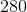?Explanation:

To solve for the greatest common factor, it is necessary to get your numbers into prime factor form. For each of your numbers, this is: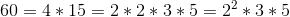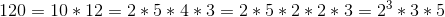Next, for each of your sets of prime factors, you need to choose the exponent for which you have the smallest value; therefore, for your values, you choose: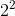Taking these together, you get: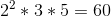### Example Question #1 : How To Find The Common Part Of A Venn Diagram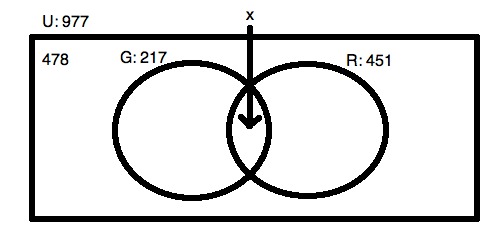For the Venn Diagram pictured above, what is the valuefor the overlap of the two sets drawn as circles?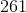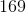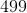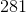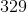Explanation:

Solving for the overlap of two sets is easy when you have all of your data. You know that the two circles added up will have to equal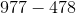or. This is the total amount in the "universe" (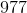minus the amount that is found outside of the two circles (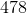).

Because the overlap happens once in each circle, you know that: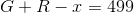Given your data, you know: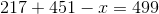Solving, this means: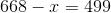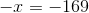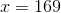### Example Question #1 : How To Find The Common Part Of A Venn Diagram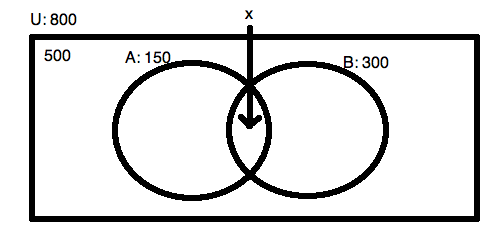For the Venn Diagram pictured above, what is the valuefor the overlap of the two sets drawn as circles?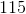Explanation:

Solving for the overlap of two sets is easy when you have all of your data. You know that the two circles added up will have to equal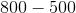or. This is the total amount in the "universe" (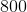minus the amount that is found outside of the two circles (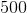).

Because the overlap happens once in each circle, you know that: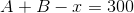Given your data, you know: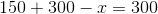Solving, this means:. The overlap is the whole of circle!

### Example Question #11 : Data Analysis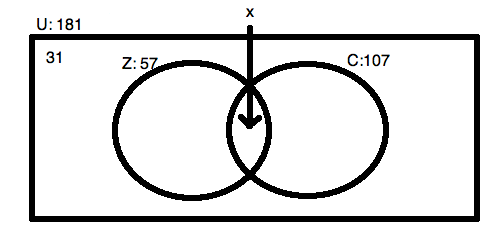For the Venn Diagram pictured above, what is the valuefor the overlap of the two sets drawn as circles?Explanation:

Solving for the overlap of two sets is easy when you have all of your data. You know that the two circles added up will have to equal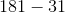or.  This is the total amount in the "universe" (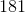minus the amount that is found outside of the two circles ().

Because the overlap happens once in each circle, you know that: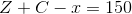Given your data, you know: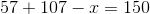Solving, this means: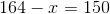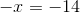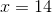### Example Question #12 : Data Analysis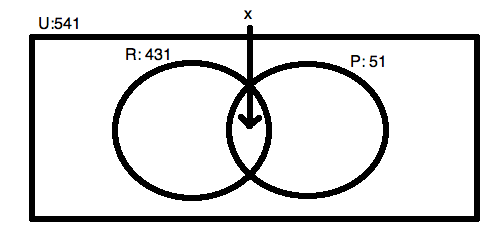For the Venn Diagram pictured above, what is the valuefor the overlap of the two sets drawn as circles?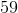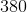No answer is possible.No answer is possible.

Explanation:

Do not be tricked by this question! In order to solve for the overlap, you need to know the amount that is in the area outside of the circles but still inside the universal box area! You cannot figure out the answer without knowing this fact; therefore, you must select "No answer is possible."  We know that the two circles do not exhaust the universe because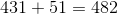. This is not large enough to fill the complete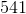. If it were, you would know that the overlap is.

### Example Question #1 : Tables

The beverage menu from a coffeehouse reads as follows: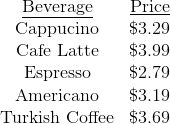Eight friends go out for drinks. Three order cappucino, two order espresso, one orders Americano, one orders Turkish coffee, and one orders cafe latte. One of the eight agrees to leave the entire amount of the tip, which is to be 20% of the check. How much will be the amount of the tip (round to the nearest dime)?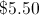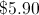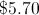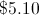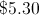Explanation:

The costs of the beverages will be as follows: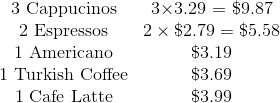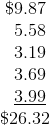20% of this is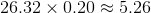, which rounds to \$5.30.

### Example Question #1 : Tables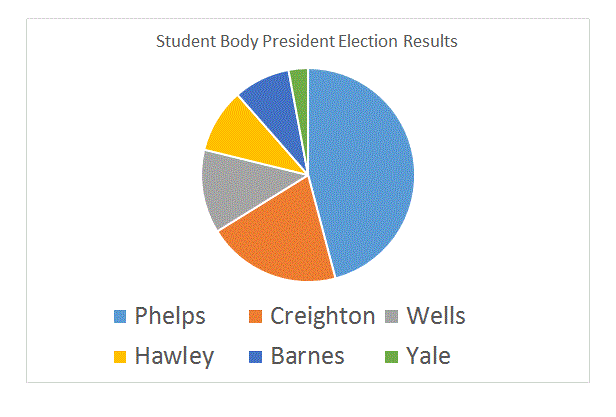Refer to the above circle graph. Which of these quantities is greater?

(a) The number of votes won by Hawley

(b) The number of votes won by Wells

(a) is greater

(a) and (b) are equal

(b) is greater

It is impossible to tell from the information given

(b) is greater

Explanation:

The gray portion of the circle graph represents Wells; the yellow portion represents Hawley. The gray portion is larger, so Wells won more votes, making (b) greater.

### Example Question #1 : TablesRefer to the above circle graph. Which of these quantities is greater?

(a) The number of votes won by Creighton

(b) The number of votes won by either Barnes or Yale

(a) is greater

(a) and (b) are equal

(b) is greater

It is impossible to tell from the information given

(a) is greater

Explanation:

The size of the orange portion of the circle, which represents Creighton, is larger than the combined sizes of the dark blue and green portions, which represent Barnes and Yale, respectively. This makes (a) the greater quantity.

### Example Question #1 : How To Find The Answer From A Table

The following is a portion of the menu at Moonbucks Coffee: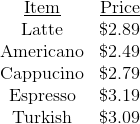On this particular day, Moonbucks offers a 20% discount if four drinks of the same kind are purchased.

Which is the greater quantity?

(a) The price of four Turkish coffees

(b) The price of two Cafe Americanos and two lattes

(b) is greater

(a) and (b) are equal

It is impossible to tell from the information given

(a) is greater

(b) is greater

Explanation:

(a) The four Turkish coffees cost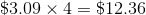before the discount. The discount is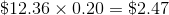, so the price after discount is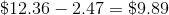.

(b) The two Cafe Americanos cost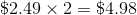; the two lattes cost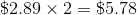. The total cost is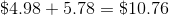.

This makes (b) the greater quantity.

### Example Question #2 : How To Find The Answer From A TableRefer to the above circle graph. Which of these quantities is greater?

(a) The number of students who voted

(b) The number of students who did not vote

It is impossible to tell from the information given

(b) is greater

(a) is greater

(a) and (b) are equal